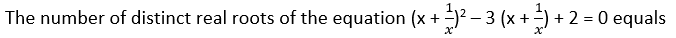# The number of distinct real roots | Quantitative Aptitude - CAT Quadratic Equation

## CAT 2020 - Slot 1 - Quantitative Aptitude - Quadratic Equation - Question 11 - The number of distinct real roots

Q. 11:x + 1/x = y

y2 – 3y + 2 = 0

y ≥ 2 & y ≤ – 2

(y – 1) (y – 2) = 0

y = 1(Not possible) or y = 2

Now, x + 1/x = 2

This has only one option x = 1. So, only one real root.

### Past Year Question Paper & SolutionsCounselling Session
By IIM Mentor

#### Free Material Area